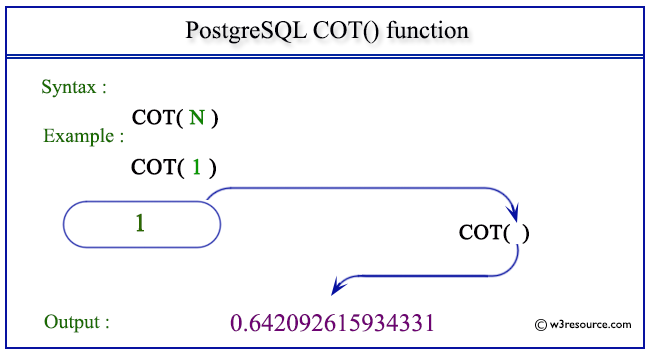# PostgreSQL COT() function

## COT() function

The PostgreSQL cot() function is used to return the cotangent of a given argument.

Syntax:

```cot(a)
```

PostgreSQL Version: 9.3

Pictorial presentation of PostgreSQL COT() functionExample 1: PostgreSQL COT() function

Code:

``````SELECT COT(0) AS "Cot (0)";
```
```

Sample Output:

``` Cot (0)
----------
Infinity
(1 row)
```

Example 2: PostgreSQL COT() function

Code:

``````SELECT COT(1) AS "Cot (1)";
```
```

Sample Output:

```      Cot (1)
-------------------
0.642092615934331
(1 row)
```

Example 3: PostgreSQL COT() function

Code:

``````SELECT COT(-1) AS "Cot (-1)";+
```
```

Sample Output:

```      Cot (-1)
--------------------
-0.642092615934331
(1 row)
```

Previous: COS function
Next: DEGREES function

﻿